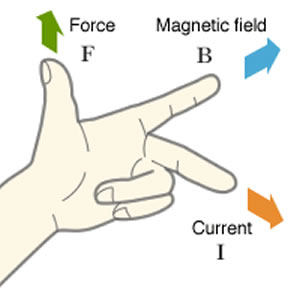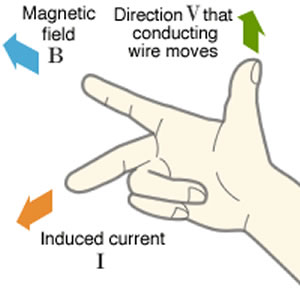# Difference between Left Hand Rule and Right Hand Rule

##### Key Difference: The Left Hand Rule and Right Hand Rule are types of visual mnemonics that were developed by John Ambrose Fleming in the late 19th century. They are used to show the direction of the motion, field and current. The Left Hand Rule applies to electric motors, while the Right Hand Rule applies to generators.The Left Hand Rule and Right Hand Rule are types of visual mnemonics. Mnemonics is a learning technique that helps one to remember certain information, such as the use of knuckles to remember which months of the year have 31 days. Similarly, the left hand rule and right hand rule are visual mnemonics that were developed by John Ambrose Fleming in the late 19th century. They are used to show the direction of the motion, field and current. They are particularly effective in finding out the direction of one, if the other two are known.

The Left Hand Rule is a simple way of working out the direction of motion in an electric motor. This rule helps one to understand and remember in which direction is the motion in an electric motor. When the left hand is held in a particular manner, the thumb and the first two fingers on the hand can represent the three mutually orthogonal axes.

In order to use the left hand rule, the left hand must be positioned in a particular manner so as to make a K. To do that, the thumb must be pointed up, whereas the first two fingers must be spread out, the last two fingers must be closed in a particle fist, i.e. held to the palm. When the thumb and first two fingers represent a K with the two fingers held at a 90 deg angle, it can show this:

• The Thumb represents the direction of the Thrust on the conductor / Motion of the Conductor.
• The Fore / First finger represents the direction of the magnetic Field
• The Centre finger represents the direction of the Current

While, the left-hand rule is used to indicate the direction of motion in an electric motor, Fleming's right-hand rule can be used for generators. The right hand rule shows the direction of induced current when a conductor moves in a magnetic field.In order to use the right hand rule, the right hand must be held in a similar manner to the left hand, i.e. forming a K. Hence, the right hand is essentially a mirror image of the left hand. Another manner to explain this is to hold the right hand with the thumb, first finger and second finger mutually perpendicular to each other (at right angles). When in this position, it can be used to remember:

• The Thumb represents the direction of Motion of the conductor.
• The First finger represents the direction of the Field. (north to south)
• The Second finger represents the direction of the induced or generated Current (the direction of the induced current will be the direction of conventional current; from positive to negative).

Another way to remember the right hand rule is remembering the “FBI” acronym. The "FBI" acronym stands for Force (or otherwise motion), B as the magnetic field sign and I as the current. The subsequent letters correspond to subsequent fingers, counting from the top. Thumb is F; First finger is B; Second finger is I.

Comparison between Left Hand Rule and Right Hand Rule:

 Left Hand Rule Right Hand Rule Invented by John Ambrose Fleming John Ambrose Fleming Used for Electric Motors Generator Purpose The direction of motion in an electric motor The direction of induced current when a conductor moves in a magnetic field. Thumb The Thumb represents the direction of the Thrust on the conductor / Motion of the Conductor. The Thumb represents the direction of Motion of the conductor. First / Fore Finger The Fore finger represents the direction of the magnetic Field The First finger represents the direction of the Field. (north to south) Second / Middle / Center Finger The Centre finger represents the direction of the Current The Second finger represents the direction of the induced or generated Current (the direction of the induced current will be the direction of conventional current; from positive to negative).

Image Courtesy: daido-electronics.co.jp